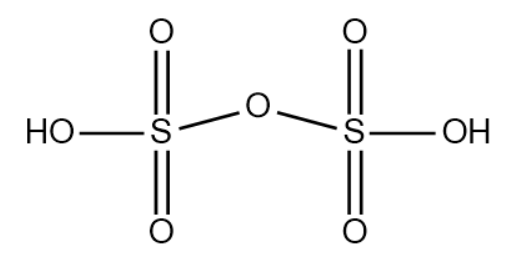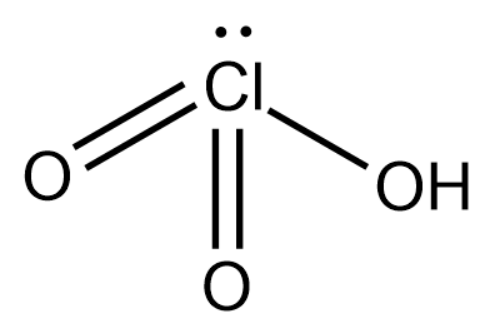# Draw structure of the following:(a) ${{H}_{2}}{{S}_{2}}{{O}_{7}}$(b) $HCl{{O}_{3}}$Verified
178.2k+ views
Hint: Identify the compounds given in the question with their common name/IUPAC name. Now identify the central atom in each of the compounds and calculate the oxidation number for them as well. With the help of oxidation state, you can determine the number of bonds that can be made around the atom and finally draw the complete expanded structure of the two compounds.

(a) Compound can be identified as pyro sulfuric acid. The common name for the compound is fuming sulphuric acid. It is formed by the reaction between concentrated sulfuric acid and sulfur trioxide.
We will now calculate the oxidation state of sulfur in pyro sulfuric acid.
Oxidation state of O = -2
Oxidation state of H = 1
Oxidation state of S = 0 - [(2 x 1) + (7 x (-2)] / 2 = 6
Based on this we will construct the diagram of pyro sulfuric acid.(b) Compound can be identified as chloric acid. It is a colorless liquid commonly used to accelerate the burning of combustible materials and can almost ignite on further contact.
We will now calculate the oxidation state of chlorine in chloric acid.
Oxidation state of O = -2
Oxidation state of H = 1
Oxidation state of Cl = 0 - [(1 x 1) + (3 x (-2)] = 5
Based on this we will construct the structure of chloric acid.Note: In the calculation of oxidation state of sulfur in pyro sulfuric acid, we observe that the oxidation state of S is 6. However, by calculation we get the answer as 12. This is because there are two sulfur atoms present for 1 molecule of the compound. This is the reason why the oxidation state found by calculation must be divided by 12 to arrive at the correct oxidation state.